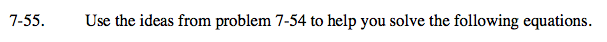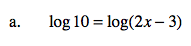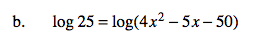Home > A2C > Chapter 7 > Lesson 7.1.4 > Problem7-55

7-55.
1. Use the ideas from problem 7-54 to help you solve the following equations. Homework Help ✎

1. log10 = log(2x − 3)

2. log 25 = log(4x2 − 5x − 50)If log a = log b, then a = b.

10 = 2x − 3See part (a).